# #4) A series RCL circuit contains a 48.0-? resistor, a 1.70-?F capacitor, and a 4.20-mH inductor....

#4) A series RCL circuit contains a 48.0-? resistor, a 1.70-?F capacitor, and a 4.20-mH inductor. When the frequency is 2575 Hz, what is the power factor of the circuit?

XC=1/(2*3.14*2575*1.7*10^-6)=36.38 ohm

XL=2*3.14*2575*(4.2*10^-3)=67.92 ohm

R=48 ohm

Z=(R^2+(XL-XC)^2)^0.5=57.43 ohm

power factor=R/Z=0.8356

XC=1/(2*3.14*2575*1.7*10^-6)=36.375ohm

XL=2*3.14*2575*(4.2*10^-3)=67.918ohm

R=48ohm

Z=(R^2+(XL-XC)^2)^0.5=57.43ohm

power factor=R/Z=0.8358

you got correct

? = 2pi*f = 2pi*2575 = 16171 rad/s

Inductive reactance XL = ?*L = 16171 * 4.20*10^-3 H = 67.91 ?

Capacitive reactance Xc = -1 / ?*C = 1/(16171 * 1.7*10^-6) = -36.37 ?

Net reactance X = 67.91 - 31.2 = 36.71 ?

Impedance angle is arctan(X/R) = tan(36.71 / 48) = 37.40 deg

Power Factor = cos(37.40) = 0.794

Power Factor =0.794

#### Earn Coin

Coins can be redeemed for fabulous gifts.

Similar Homework Help Questions
• ### A series RCL circuit contains a 25.8-Ω resistor, a 1.02-μF capacitor, and a 2.72-mH inductor. When...

A series RCL circuit contains a 25.8-Ω resistor, a 1.02-μF capacitor, and a 2.72-mH inductor. When the frequency is 2800 Hz, what is the power factor of the circuit?

• ### A radio contains a series RCL circuit with a 250 mH inductor, a 2.8 microF capacitor,...

A radio contains a series RCL circuit with a 250 mH inductor, a 2.8 microF capacitor, and a 960 Ohm resistor (the speaker). When plugged into an AC wall outlet in the US, the speakers output is 15 W. If the radio is plugged into 40.0 Hz, 180-V rms AC outlet in another country, will the speaker be louder or softer than in the US (which uses a different frequency and rms voltage)?

• ### A series AC circuit contains a resistor, an inductor of 220 mH, a capacitor of 4.20...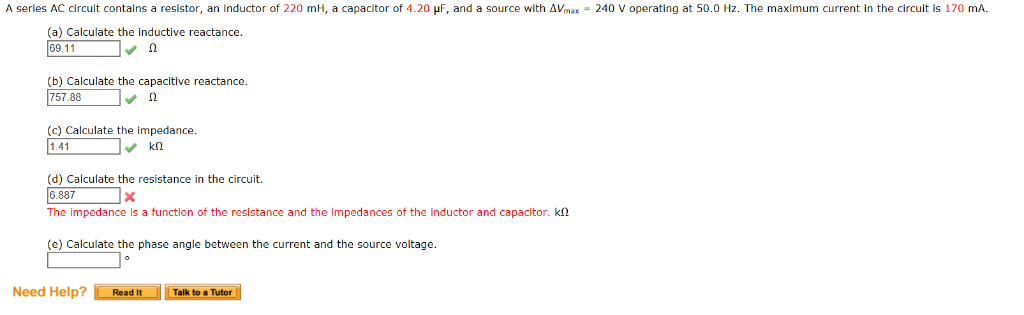A series AC circuit contains a resistor, an inductor of 220 mH, a capacitor of 4.20 ur, and a source with ΔⅤmax-240 V operating at 50.0 Hz. The maximum current in the circuit is 170 mA. (a) Calculate the inductive reactance 69.11 (b) Calculate the capacitive reactance 757.88 (c) Calculate the impedance 141 (d) Calculate the resistance in the circuit. 6.887 The impedance is a function of the resistance and the impedances of the inductor and capacitor. kΩ (e) Calculate...

• ### A series RCL circuit operating at 60.0 Hz contains a 35-W resistor and an 8.2-µF capacitor....

A series RCL circuit operating at 60.0 Hz contains a 35-W resistor and an 8.2-µF capacitor. If the power factor of the circuit is +1.00, what is the inductance of the inductor in this circuit?

• ### Fig.1 9. A series RCL circuit contains a 148- 2 resistor, a 1.50-uF capacitor, and a...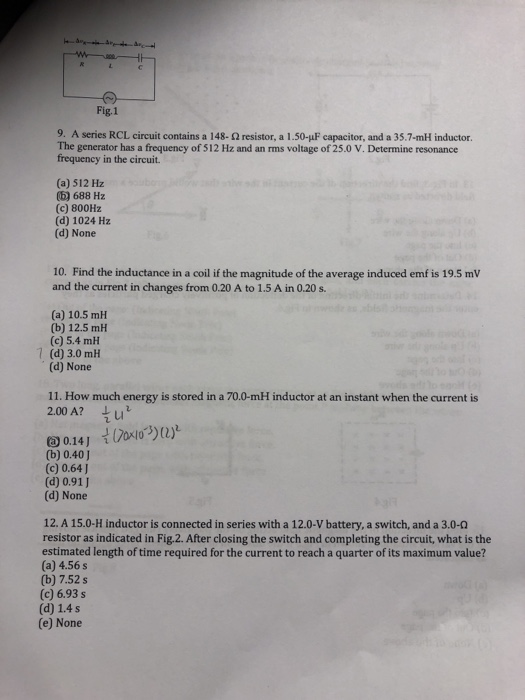Fig.1 9. A series RCL circuit contains a 148- 2 resistor, a 1.50-uF capacitor, and a 35.7-mH inductor. The generator has a frequency of 512 Hz and an ms voltage of 25.0 V. Determine resonance frequency in the circuit. (a) 512 Hz (5) 688 H2 (c) 800Hz (d) 1024 Hz (d) None 10. Find the inductance in a coil if the magnitude of the average induced emf is 19.5 mV and the current in changes from 0.20 A to 1.5...

• ### Exercise 31 A series ac circuit contains a 350 - resistor, a 20.0 - mH inductor,...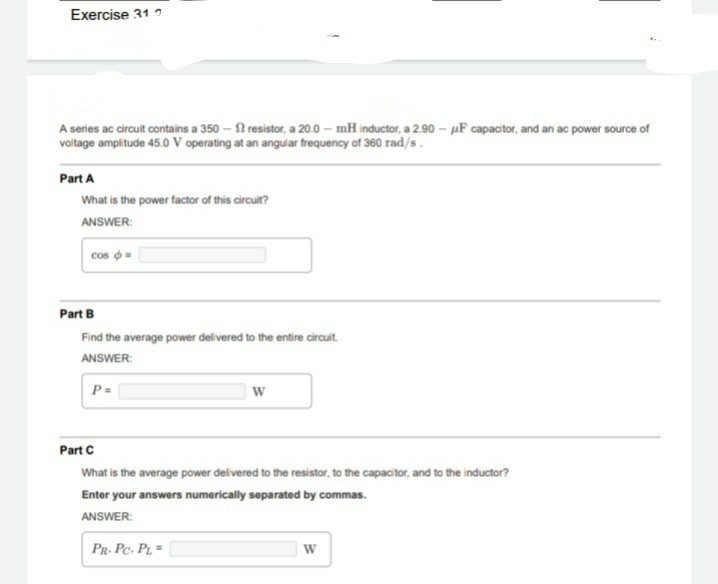Exercise 31 A series ac circuit contains a 350 - resistor, a 20.0 - mH inductor, a 2.90 - uF capacitor, and an ac power source of voltage amplitude 45.0 V operating at an angular frequency of 360 rad/s. Part A What is the power factor of this circuit? ANSWER: cos - Part B Find the average power delivered to the entire circuit ANSWER: P- w Part C What is the average power delivered to the resistor, to the capacitor,...

• ### What capacitor in series with a 100 Ohm resistor and a 20.0 mH inductor will give...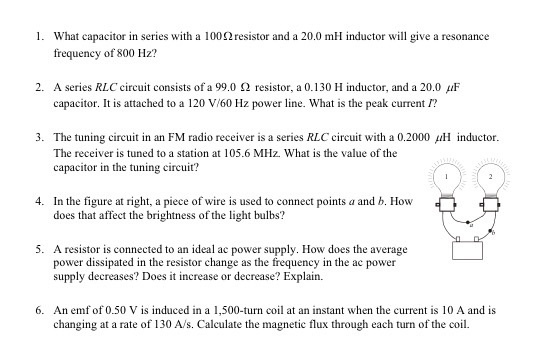What capacitor in series with a 100 Ohm resistor and a 20.0 mH inductor will give a resonance frequency of 800 Hz? A series RLC circuit consists of a 99.0 Ohm resistor, a 0.130 H inductor, and a 20.0 muF capacitor. It is attached to a 120 V/60 Hz power line. What is the peak current I? The tuning circuit in an FM radio receiver is a series RLC circuit with a 0.2000 muH inductor. The receiver is tuned to...

• ### 1) An RLC series circuit has a 40.0 ? resistor, a 3.00 mH inductor, and a...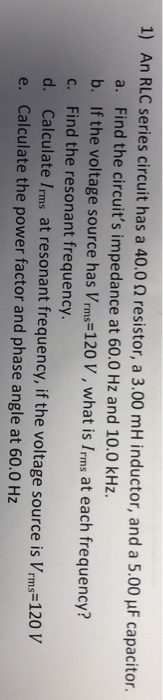1) An RLC series circuit has a 40.0 ? resistor, a 3.00 mH inductor, and a 5.00 ?F capacitor a. Find the circuit's impedance at 60.0 Hz and 10.0 kHz b. If the voltage source has Vrms 120 V, what is Irms at each frequency? c. Find the resonant frequency d. Calculate Irms at resonant frequency, if the voltage source is Vrms-120v e. Calculate the power factor and phase angle at 60.0 Hz

• ### A series AC circuit contains a resistor, an Inductor of 220 mH, a capacitor of 4.80...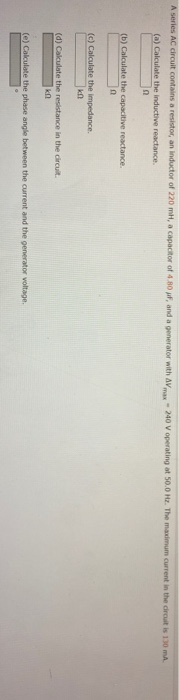A series AC circuit contains a resistor, an Inductor of 220 mH, a capacitor of 4.80 f, and a generator with Av max - 240 V operating at 50.0 Hz. The maximum current in the circuit is 130 mA (a) Calculate the inductive reactance (b) Calculate the capacitive reactance (c) Calculate the impedance kn (d) Calculate the resistance in the circuit kn (e) Calculate the phase angle between the current and the generator voltage

• ### A series AC circuit contains a resistor, an inductor of 250 mH, a capacitor of 4.50...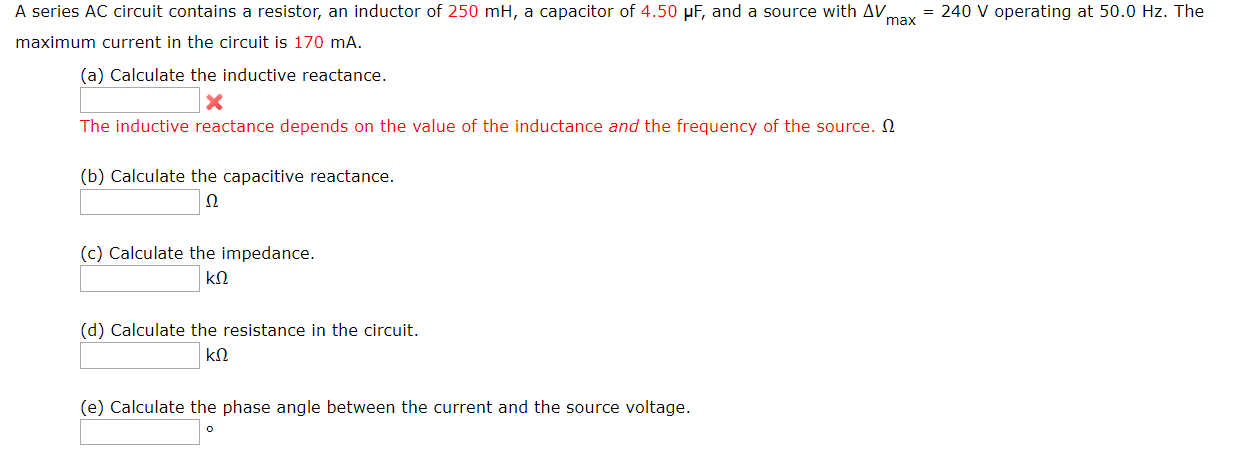A series AC circuit contains a resistor, an inductor of 250 mH, a capacitor of 4.50 uF, and a source with AV = 240 V operating at 50.0 Hz. The max maximum current in the circuit is 170 mA. (a) Calculate the inductive reactance. The inductive reactance depends on the value of the inductance and the frequency of the source. Q (b) Calculate the capacitive reactance. (c) Calculate the impedance. kn (d) Calculate the resistance in the circuit. kn. (e)...